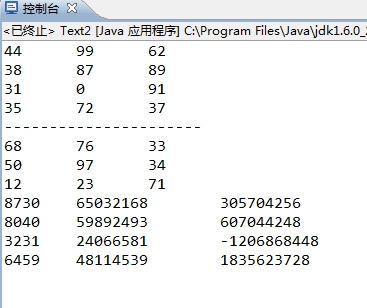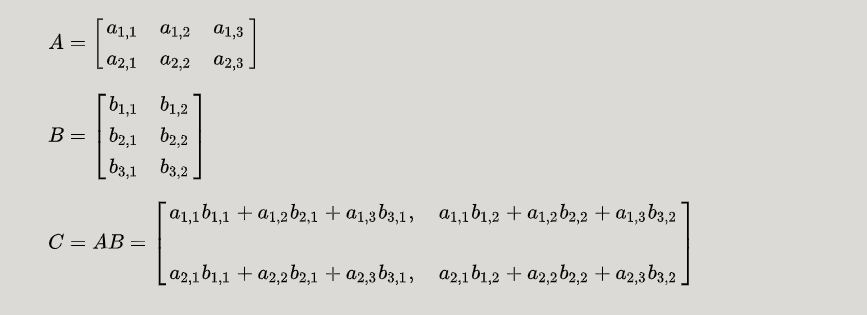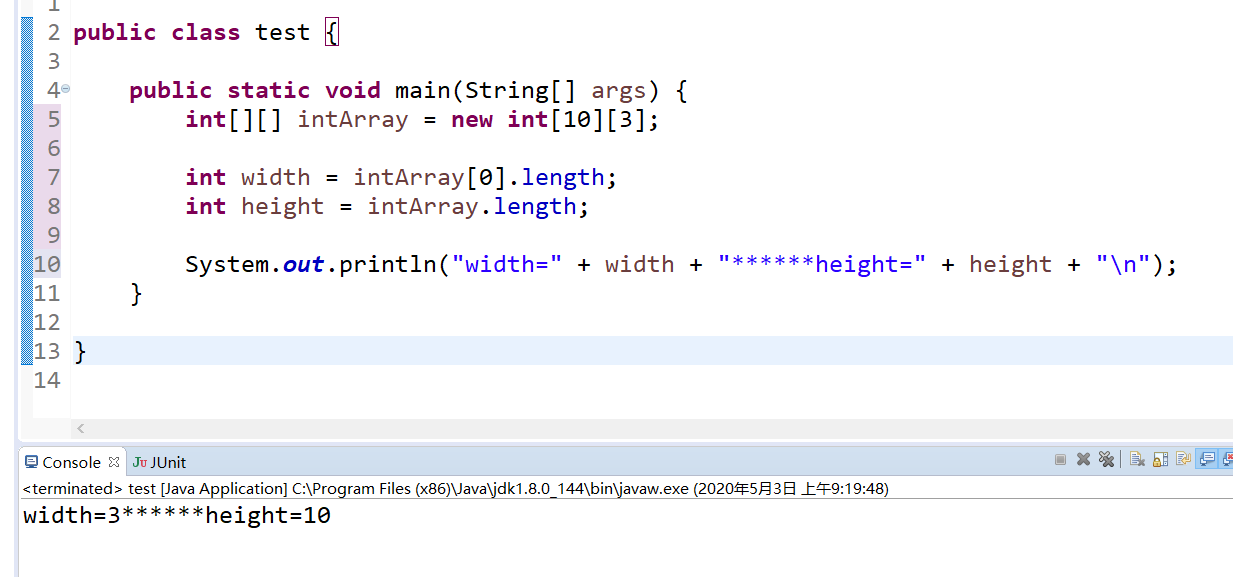• 二维数组相乘
2022-04-26 01:48:33

import java.io.InputStream;
import java.util.Scanner;
public class Main {
public static void main(String[] args) {
Matrix firstMat=Matrix.inputMatrix();
Matrix secondMat=Matrix.inputMatrix();
//display(firstMat.matrix);    display(secondMat.matrix);

Matrix productMat=firstMat.multiply(secondMat);
display(productMat.matrix);
}

//display方法：打印二维数组元素到屏幕
public static void display(int[][] array){
for(int i=0; i<array.length; i++){
for(int j=0; j<array[i].length; j++){
if(j==array[i].length-1) {
System.out.println(array[i][j]);
}
else {
System.out.print(array[i][j]+" ");
}
}
}
}
}
/*
* 编写程序题, 根据要求编写一个Java类，补充到代码中。
定义Matrix(矩阵)类，使用二维数组实现矩阵，实现两个矩阵的乘法。要求如下：

根据程序需求定义构造方法。
inputMatrix方法：从键盘读入一个矩阵，读入行数和列数，创建矩阵，然后读入元素值方法。
multiply方法：两个矩阵相乘，并返回乘积矩阵。
(两个矩阵的乘法：一个m×n的矩阵a（m,n)乘一个n×p的矩阵b（n,p)，会得到一个m×p的矩阵c（m,p)。矩阵的行数和列数自定。）
*/

class Matrix {
int row;
int column;
int[][] matrix;
static Scanner reader = new Scanner(System.in);

public static Matrix inputMatrix() {
Matrix ret = new Matrix();

ret.matrix = new int[ret.row][ret.column];

for(int i = 0; i < ret.row; i++) {
for(int j = 0; j < ret.column; j++) {

}
}
return ret;
}

Matrix multiply(Matrix mat) {
Matrix ret = new Matrix();
ret.row = row;
ret.column = mat.column;
ret.matrix = new int[row][mat.column];
for(int i = 0; i < row; i++) {
for(int j = 0; j < mat.column; j++) {
for(int k = 0; k < mat.row; k++) {
ret.matrix[i][j] += matrix[i][k] * mat.matrix[k][j];
}
}
}
return ret;
}
}

//注意三重循环使用

//很神奇厉害的地方 可以在类的方法种创建类对象

//类对象也可以作为参数  参数可以是一个类  这个参数包含类中所有的实例与方法(？即便是方法未写完？还是说只能用其中的实例  一般应该也只会用到实例)

//Matrix productMat=firstMat.multiply(secondMat); 调用类的方法

//可以有返回类型为类的方法 注意这种方法的写法

更多相关内容
• java 二维数组矩阵乘法的实现方法，需要的朋友可以参考一下
• 展开全部importjava.util.Random;publicclassText2{publicstaticvoidmain(String[]args){Matrixs3=newMatrix(4,3);Matrixs4=newMatrix(3,3);s3.printMatrix();System.out.println("------------------...

展开全部

import java.util.Random;

public class Text2 {

public static void main(String[] args){

Matrix s3 = new Matrix(4, 3);

Matrix s4 = new Matrix(3, 3);

s3.printMatrix();

System.out.println("----------------------");

s4.printMatrix();

s3.mulMatrix(s4);

s3.printMatrix();

}

public static class Matrix {

private int[][] a;

Random random = new Random();

// 构造方法e69da5e887aa3231313335323631343130323136353331333264656665

public Matrix() {

a = new int;

}

public Matrix(int n) {

a = new int[n][n];

for (int i = 0; i

for (int j = 0; j

a[i][j] = random.nextInt(100);

}

}

}

public Matrix(int n, int m) {

a = new int[n][m];

for (int i = 0; i

for (int j = 0; j

a[i][j] = random.nextInt(100);

}

}

}

public int[][] getMatrix() {

return a;

}

// 打印一个矩阵

public void printMatrix() {

for (int i = 0; i

for (int j = 0; j

System.out.print(a[i][j] + "\t");

}

System.out.println();

}

}

// 矩阵相加

public void plusMatrix(Matrix b) {

int[][] c = b.getMatrix();

int n = c.length;

int m = c.length;

if (n != a.length || m != a.length) {

System.out.println("长度不一，不能相加");

} else {

for (int i = 0; i

for (int j = 0; j

a[i][j] = a[i][j] + c[i][j];

}

}

}

}

// 矩阵相乘

public void mulMatrix(Matrix b) {

int[][] c = b.getMatrix();

int n = c.length;

int m = c.length;

if (n != a.length) {

System.out.println("第一个矩阵的列数不等于第二个矩阵的行数，不能进行乘法运算");

} else {

for (int i = 0; i

for (int j = 0; j

for (int k = 0; k

a[i][j] += (a[i][k] * c[k][j]);

}

}

}

}

}

}

}展开全文• 若A为NxM阶矩阵 B为MxP阶矩阵，二者相乘之后的矩阵则为NXP阶矩阵 运算过程： 思路 计算并显示出来这个结果 我们则需要 一个计算方法 和 一个显示方法 因此主函数可以写的相当之简单 ， 键入想要求解的矩阵的数值 ...

# 知识回顾

若A为NxM阶矩阵 B为MxP阶矩阵，二者相乘之后的矩阵则为NXP阶矩阵
运算过程：# 思路

• 计算并显示出来这个结果 我们则需要 一个计算方法 和 一个显示方法
• 因此主函数可以写的相当之简单 ， 键入想要求解的矩阵的数值 然后调用两种方法即可

## 显示方法

• 只需要循环显示出行列值然后分行即可

## 计算方法

设进行矩阵乘法的两个矩阵为a 3x3型矩阵和b 3x2型矩阵

• 我们在运用数学思维计算矩阵乘法时首先计算的便是
z = a*b+a*b+a*b
转换成代码则可想到 矩阵可以利用二维数组来实现
下标的改变以及算术累加可以用for循环来实现
• 在此之上我们可以再思考
z计算过后我们将会继续计算z 而列下标的改变则可再次利用循环来实现
以此类推
• 矩阵第一行的数值计算过后 我们要转为求解第二行的数值 这时则可再次利用循环来实现行的改变

因此 二维数组实现矩阵乘法的基本思路就是 利用嵌套循环来实现数值的累加计算，矩阵列以及行的变化。

以下为实现代码：

public class Example4_04{
public static void main(String[] args){
int[][] a={{-4 , 5 , -7},
{2, -3 , -4},
{3 , 4 , 5}};
int[][] b={{1 , 2},
{5 , 6},
{-7 , 9},};
int[][] result = mul(a,b);
print(result);
}
//mul返回值的类型是一个二维数组
static int[][] mul(int[][] x , int[][] y)
{
int i , j , k;//循环控制变量
//申请结果数组的空间
//行长度为x数组的行长度，列长度为y数组第一行的行长度
int[][] z=new int[x.length][y.length];
for(i = 0 ; i < x.length ; i++)
{	for(j = 0 ; j < y.length ; j++)
{
z[i][j] = 0;
for(k = 0 ; k < x.length ; k++)
z[i][j] = z[i][j] + x[i][k] * y[k][j];
}
}
return z;
}
static void print(int[][] x){
int i , j;
for(i = 0 ; i < x.length ; i++){
for(j = 0 ; j < x[i].length ; j++)
System.out.printf("%5d",x[i][j]);
System.out.println();
}
}
}


展开全文算法 矩阵
• 可以看到intArray.length这样可以是返回高度10的意思，也就是第一列数组的长度，而intArray.length被用来返回宽度，如果想知道数组总元素个数，可以用这个两个相乘。 ...可以看到intArray.length这样可以是返回高度10的意思，也就是第一列数组的长度，而intArray.length被用来返回宽度，如果想知道数组总元素个数，可以用这个两个相乘。

展开全文• import java.util.*; //由于数组长度不确定，因此我用ArrayList实现 public class ArrayMatrix { public static void main(String[] args) { Scanner input = new Scanner(System.in); System.out.pr.list 矩阵
• 前言 本篇文章主要总结了矩阵的常见运算，以及Java中利用二维数组进行运算的实现 1.矩阵运算 1.矩阵加法 运算前提：多个矩阵的行数和列数相同，我们称它们为同型矩阵，只有是同型矩阵才能进行加减运算。 运算规则：...程序人生 开发语言 矩阵 算法
• ## 一维数组相乘

千次阅读 2020-03-12 22:41:28
两个一维数组相乘并输出 package 乘法; import java.util.Scanner; public class Chengfa { public static int[] Cf(int[] a,int[]b) { int c[]=new int[a.length]; if(a.length!=b.length) { System.out.println(...
• java二维数组的个数Here, We have to find the multiplication of the two matrix by moving forward direction in row major (row by row) order in one matrix and by backward direction in row major order in ...
• 之前看到有人在网上询问如何用Java多多线程实现二维数组的乘法。于是回去仔细思考了一下，实现出来分享一下。多线程
• 二维数组 二维数组简单来说就是一个由行和列组成的一个表格，也就是数学中的矩阵。我们在这个矩阵中访问元素的话，是根据元素的行角标和列角标所确定的。 那么具体是在内存中如何存储的呢？ 无论是二维数组，...
• 二维数组 就是一个由行和列组成的一个表格而已，矩阵Matrix。 我们在这个矩阵中访问元素的话，是根据元素的行角标和列角标所确定的。 1、那么对于二维数组是如何存储的呢？ 无论是二维数组，还是多维数组，...
• protected void Page_Load(object sender, ...}//floor向下取整//ceiling 向上取整//开平方就用Math.Sqrt, 开n次方:Math.Pow(double, 1/n)//一维数组存储二维矩阵N*N，得到行和列// 行：i / n ；列：i % npriv...
• //矩阵相乘得到新的二维数组。 public class MatrixMultiply { public static void main(String[] args) { int i, j, k; int[][] a = new int; int[][] b = { { 1, 5, 2, 8 }, { 5, 9, 1
• 主要为大家详细介绍了Java构建乘积数组的方法，具有一定的参考价值，感兴趣的小伙伴们可以参考一下
• 文章目录线性代数中的矩阵相乘定义注意事项代码逻辑分析代码实现运行结果 线性代数中的矩阵相乘 定义 设A为 的矩阵，B为 的矩阵，那么称 的矩阵C为矩阵A与B的乘积，记作 ，其中矩阵C中的第 行第 列元素可以表示为： ...
• import java.util.Arrays; /** * @Author kinmon * @Date 2020/7/15 14:44 * @Version 1.0 * 1. 输入一个 m*n的矩阵 和一个n*p的矩阵，并初始化, 要求打印出两个矩阵的乘积。 * 1、当矩阵A的列数（column）...
• 分别定义两个二维数组用来存放两个矩阵，矩阵A的行号和矩阵B的列号决定矩阵C的行列号；矩阵A的列号和矩阵B的行号相同者进行乘法操作。 现在暂且不考虑设置矩阵相乘的规则，就弄个最简单的矩阵相乘 package juzhen...矩阵
• 包括基本数据类型和引用数据类型数组长度确定下来就不能更改创建数组对象会在内存中开辟一段连续的空数组的概述数组名下标或者索引元素数组长度数组有序排列的通过下标进行管理数组长度就是元素个数数组本身属于引用...
• int[][] matrixMultiply(int[][] matrix1, int[][] matrix2) { if (matrix1 == null || matrix2 == null) { return null; } if (matrix1.length != matrix2.length) { return null; } ...算法
• packagearrayList;/*** 矩阵的集中运算法则：求和，求积，求逆矩阵，转置矩阵......*@...*@parama 矩阵(二维数组)*@paramb 矩阵(二维数组)*@return返回一个矩阵(二维数组)*/public static int[][] add(int [][] a,i...
• import java.util.Random; import java.util.Scanner; public class 矩阵运算{ public static void main(String args[]){ Random ran=new Random(); int h1 =0; int l1 =0; int h2=0; int l2=0; for(int i = 0;i<...
• 使用二维数组实现Matrix(矩阵)： 1. 定义Matrix(矩阵)类，要求如下： a) 变量：matrix(int型二维数组)，row(行数)，column(列数)； b) 方法：实现两个矩阵的乘法，所有方法将返回操作后的结果矩阵。(两个矩阵的...矩阵
• 前面我们学习了一位数组，在讲解到八皇后的问题时，我们也用到了二位数组，那么什么是二维数组呢？相信大家都应该学过二维矩阵如： 其实二维数组就是一个二维的矩阵，矩阵的行列对应的位置就是二维数组每个元素...矩阵的转置
• 目的：掌握二维数组的基本使用方法。 要求：定义两个3*3数组，使用静态初始化的方法设置数组的元素值分别为： 1,2,3 4,5,6 7,8,9 和 0, 2, 4 1.1, 4.3, 5.2 1, 4.5, 2.2 要求编写一个类，类的方法如下： (1) 编写两...
• 从图 1 可以看到，相乘后的矩阵的行数等于第一个矩阵的行数，列数等于第个矩阵的列数。 编程要求 仔细阅读右侧编辑区内给出的代码框架及注释，在 Begin-End 间编写程序代码，给定以下两个矩阵，求它们的乘积。 ...矩阵
• 然而直接通过复制粘贴测试用例输入时，经调试发现程序在接收第数组的行数和列数时，没有读到数据，而是停下，等待输入。 自己试了半天无果，想知道是哪里出了问题，希望能得到解答，谢谢！！ **测试数据：...
• 利用二维数组实现一个矩阵类：Matrix。要求提供以下操作：(1)set(introw,intcol,doublevalue)：将第row行第col列的元素赋值为value；(2)get(introw，intcol)：取第row行第col列的元素；(3)width()：返回矩阵的列数；...
• 1:二维数组(理解)(1)元素是一维数组的数组。(2)格式：A:数据类型[][]数组名= new数据类型[m][n];B:数据类型[][]数组名= new数据类型[m][];m表示这个二维数组有多少个一维数组这一次没有直接给出一维数组的元素个数，......

# java二维数组相乘java 订阅